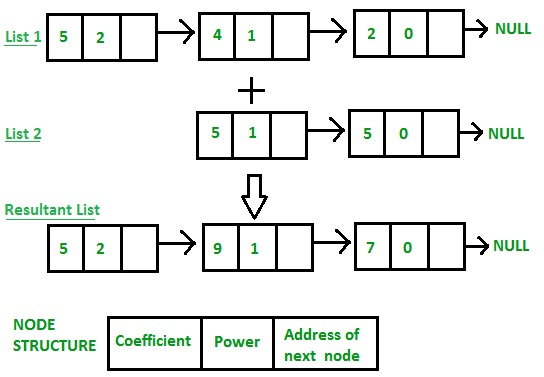# Cpp programming-Compare two strings represented as linked listsGiven two linked lists, represented as linked lists (every character is a node in linked list). Write a function compare() that works similar to strcmp(), i.e., it returns 0 if both strings are same, 1 if first linked list is lexicographically greater, and -1 if second string is lexicographically greater.

Examples:

```Input: list1 = g->e->e->k->s->a
list2 = g->e->e->k->s->b
Output: -1

Input: list1 = g->e->e->k->s->a
list2 = g->e->e->k->s
Output: 1

Input: list1 = g->e->e->k->s
list2 = g->e->e->k->s
Output: 0

```

c++ programming

``````// C++ program to compare two strings represented as linked
// lists
#include<bits/stdc++.h>
using namespace std;

struct Node
{
char c;
struct Node *next;
};

// Function to create newNode in a linkedlist
Node* newNode(char c)
{
Node *temp = new Node;
temp->c = c;
temp->next = NULL;
return temp;
};

int compare(Node *list1, Node *list2)
{
// Traverse both lists. Stop when either end of a linked
// list is reached or current characters don't match
while (list1 && list2 && list1->c == list2->c)
{
list1 = list1->next;
list2 = list2->next;
}

//  If both lists are not empty, compare mismatching
//  characters
if (list1 && list2)
return (list1->c > list2->c)? 1: -1;

// If either of the two lists has reached end
if (list1 && !list2) return 1;
if (list2 && !list1) return -1;

// If none of the above conditions is true, both
// lists have reached end
return 0;
}

// Driver program
int main()
{
Node *list1 = newNode('g');
list1->next = newNode('e');
list1->next->next = newNode('e');
list1->next->next->next = newNode('k');
list1->next->next->next->next = newNode('s');
list1->next->next->next->next->next = newNode('b');

Node *list2 = newNode('g');
list2->next = newNode('e');
list2->next->next = newNode('e');
list2->next->next->next = newNode('k');
list2->next->next->next->next = newNode('s');
list2->next->next->next->next->next = newNode('a');

cout << compare(list1, list2);

return 0;
}``````

Output:

`1`